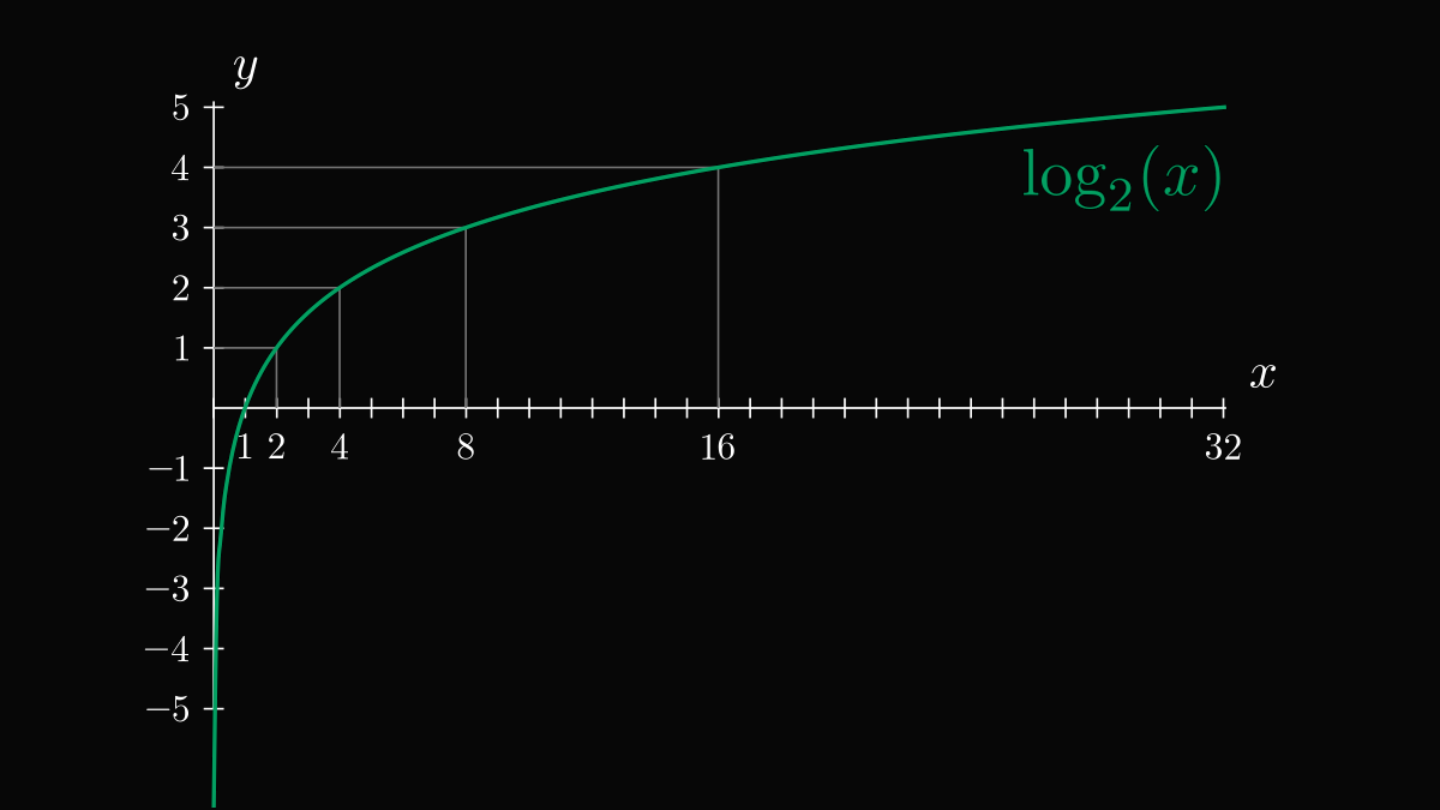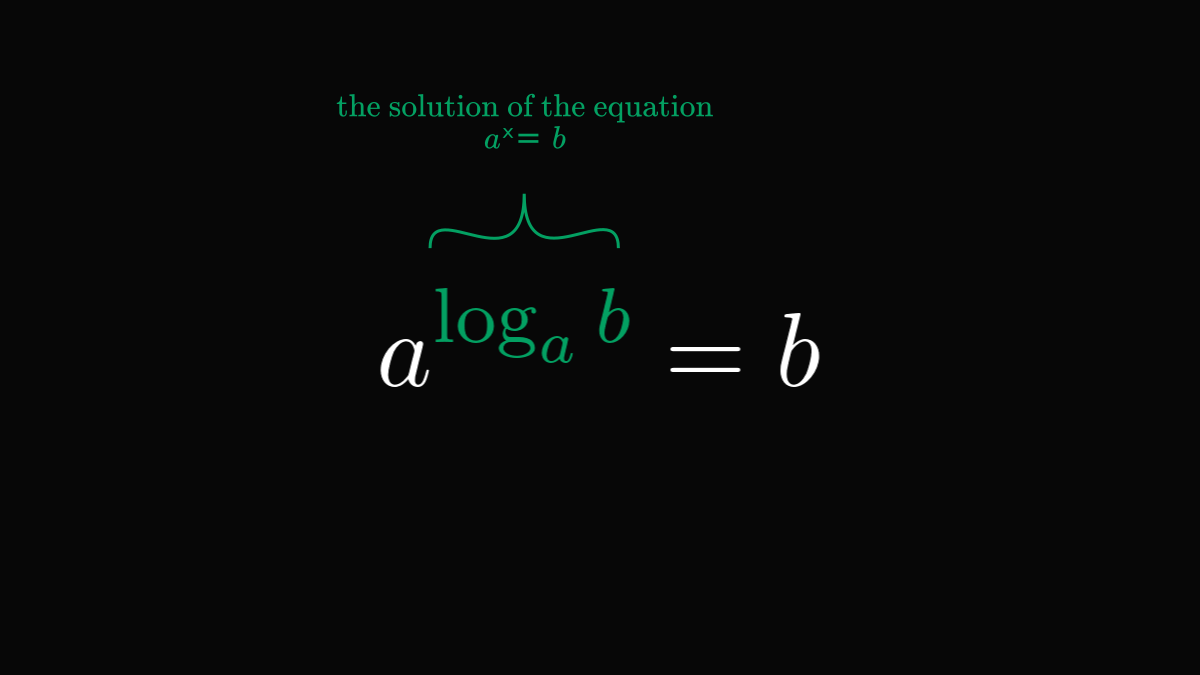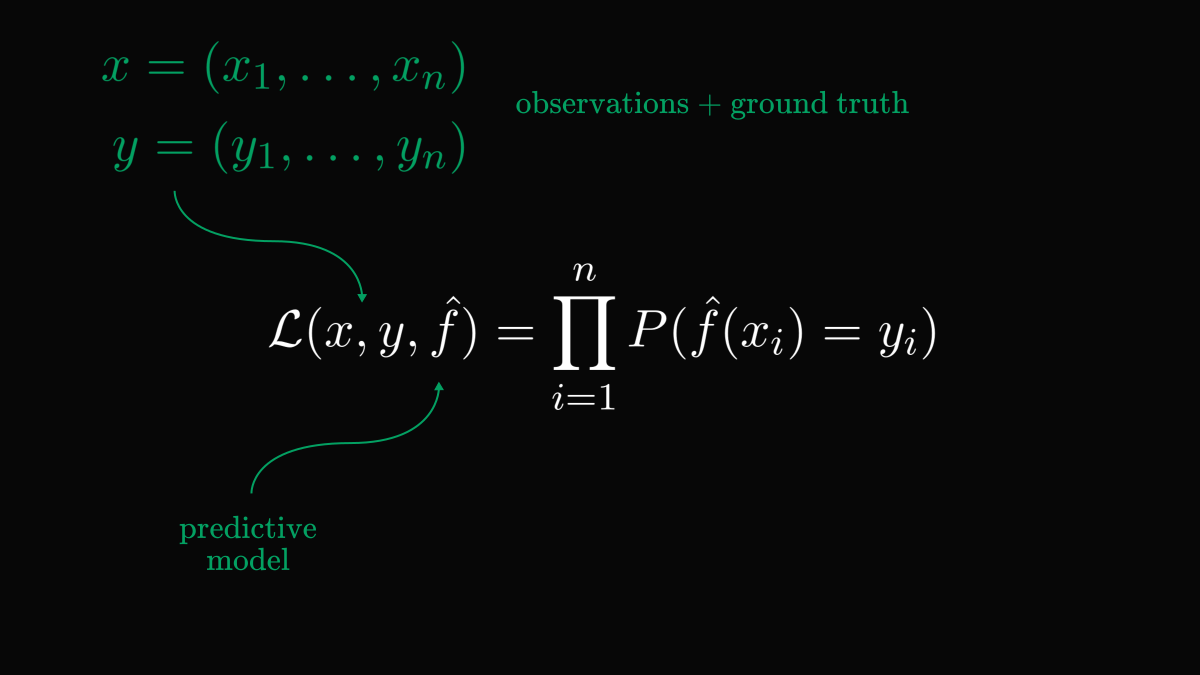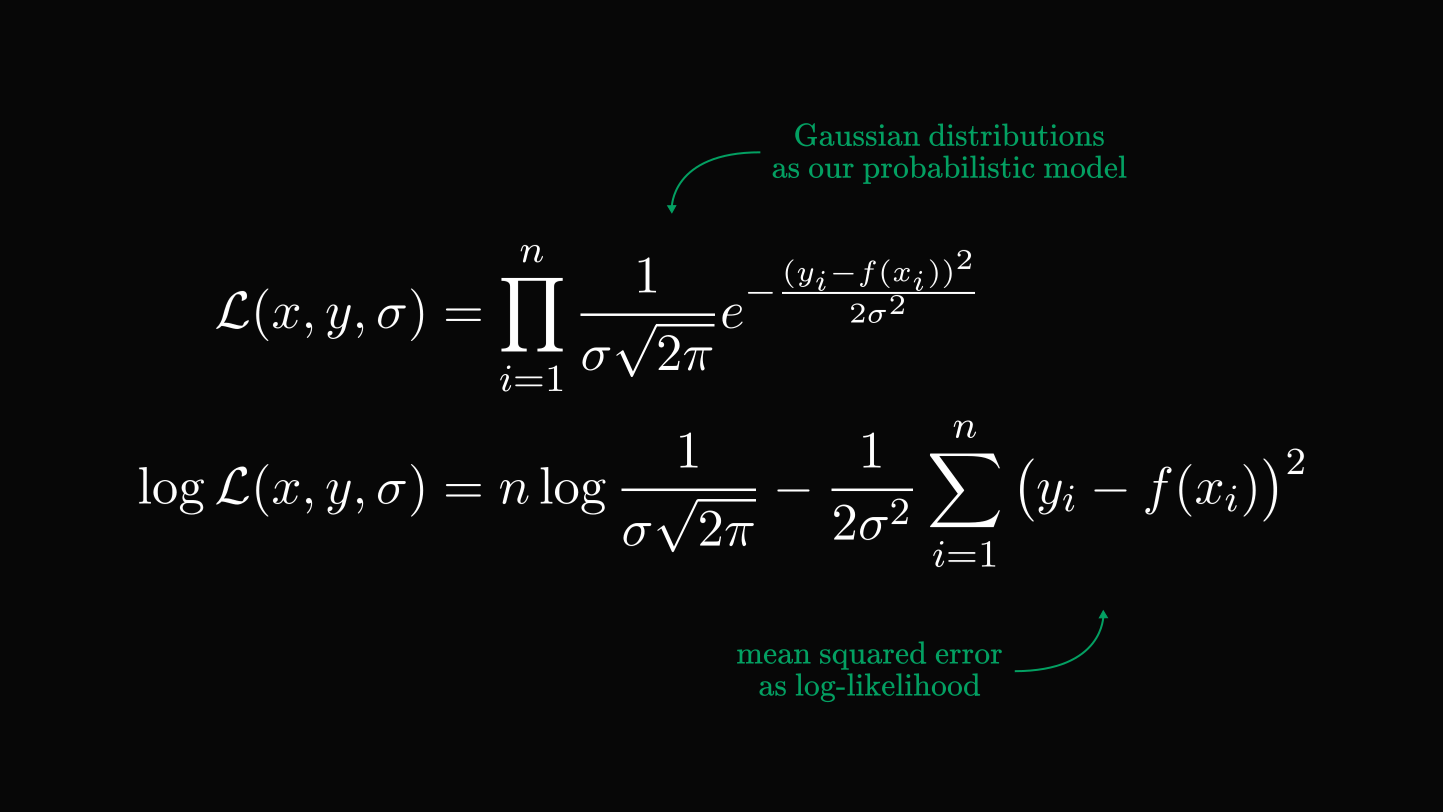# The one reason we love logarithms in machine learningThere is one big reason we love the logarithm function in machine learning.

Logarithms help us reduce complexity by turning multiplication into addition. You might not know it, but they are behind a lot of things in machine learning.

First, let's start with the definition of the logarithm. The base $a$ logarithm of $b$ is simply the solution of the equation $a^x = b$.Despite its simplicity, it has many useful properties that we take advantage of all the time.

You can think of the logarithm as the inverse of exponentiation. Because of this, it turns multiplication into addition:

$\log(xy) = \log(x) + \log(y).$

(The base of a logarithm is often assumed to be a fixed constant. Thus, it can be omitted.) Exponentiation does the opposite: it turns addition into multiplication.

Why is the property $\log(xy) = \log(x) + \log(y)$ useful? Because we can use it to calculate gradients and derivatives!

Training a neural network requires finding its gradient. However, lots of commonly used functions are written in terms of products.

As you can see, this complicates things:

\begin{align*} (f g)^\prime &= f^\prime g + f g^\prime \\ (fgh)^\prime &= f^\prime gh + fg^\prime h + fgh^\prime \\ &\vdots \end{align*}

By taking the logarithm, we can compute the derivative as it turns products into sums:

\begin{align*} \Big( \log f_1 \dots f_n \Big)^\prime &= \bigg( \sum_{i=1}^{n} \log f_i \bigg)^\prime \\ &= \sum_{i=1}^{n} \Big( \log f_i \Big)^\prime. \end{align*}

This method is called logarithmic differentiation. One example where this is useful is the maximum likelihood estimation.

Given a set of observations and a predictive model, we can write this in the following form.Believe it or not, this is behind the mean squared error!Every time you use this, logarithms are working in the background.